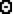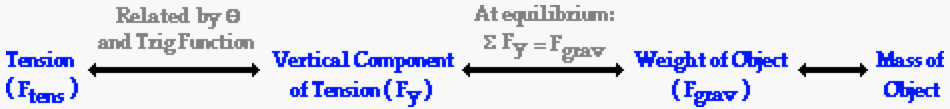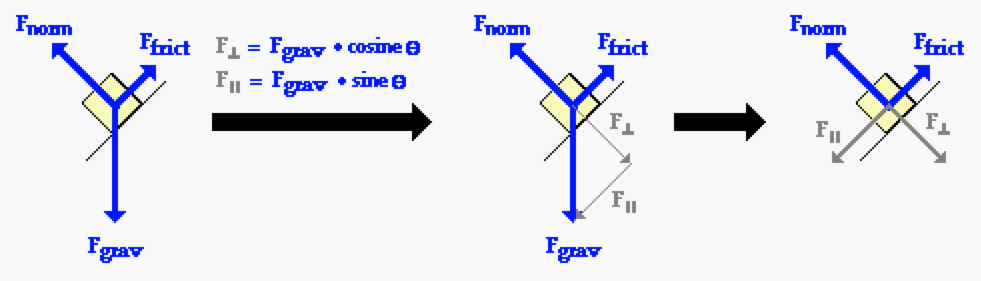# Mechanics: Vectors and Forces in Two-Dimensions

## Vectors and Forces in 2-D: Problem Set Overview

This set of 27 problems targets your ability to determine the vector sum of two or more forces (which are not at right angles to each other), analyze situations in which forces are applied at angles to the horizontal to move an object along a horizontal surface, analyze equilibrium situations to determine an unknown quantity, and to analyze the motion of objects along an inclined plane. The more difficult problems are color-coded as blue problems.

## Vector Addition and Vector Components

Two or more vectors can be added together in order to determine the resultant vector. The resultant vector is simply the result of adding two or more vectors. Vectors which make right angles to one another are easily added using the Pythagorean theorem; Trigonometric functions can be used to determine the direction of the resultant vector. Vectors which are not at right angles to each other can be resolved into components which lie along the east-west and north-south coordinate axes. Sine and cosine functions can be used to determine these components. Once all components have been determined, they can be simplified into a single east-west and a single north-south vector; then the Pythagorean theorem and trigonometric functions can be used to determine the magnitude and direction of the resultant vector.

## Counter-Clockwise Convention and Vector Components

A vector which is directed at angle to one of the coordinate axes is said to have components directed along the axes. These components describe the effect of the vector in the direction of the axes. The direction of a vector is often expressed using the counter clockwise (CCW) from east convention. By such a convention, the direction of a vector is represented as the counter-clockwise angle of rotation which the vector makes with due East. When this convention is used, the components of the vector along the east-west and north-south axes can be determined quite easily using the sine and cosine functions. If a vector has a magnitude of A and a direction of(by the CCW convention), then the horizontal and vertical components can be determined using the following equations:

Ax = A • cosAy = A • sin## Newton's Second Law

The acceleration (a) of objects is caused by an unbalanced or net force (Fnet). The magnitude of the acceleration is equal to the ratio of net force to mass: a = Fnet / m. Typical Newton's second law problems are centered around determining the net force, the mass or the magnitude of individual forces acting upon an object.

There are typically two types of these problems in this set of problems:

• Determine the individual force value: If the acceleration of an object is known, then the magnitude of the net force can usually be determined. This net force value is related to the vector sum of all individual force values; as such, the magnitude of an individual force can often be found if the net force can be calculated.
• Determine the acceleration value: If the values of all individual force values are known, then the net force can be calculated as the vector sum of all the forces. The mass is often stated or determined from the weight of the object. The acceleration of the object can then be found as the ratio of the net force to

## Mass-Weight Relationship

Mass is a quantity which is dependent upon the amount of matter present within an object; it is expressed in kilograms. Weight, on the other hand, is the force of gravity which acts upon an object. Being a force, weight is expressed in the metric unit as Newtons. Every location in the universe is characterized by a gravitational constant represented by the symbol g (sometimes referred to as the acceleration of gravity). Weight (or Fgrav) and mass are related by the equation: Fgrav = m • g.

## Friction Force

An object which is moving (or even attempting to move) across a surface encounters a force of friction. Friction force results from the two surfaces being pressed together closely, causing intermolecular attractive forces between molecules of different surfaces. As such, friction depends upon the nature of the two surfaces and upon the degree to which they are pressed together. The friction force can be calculated using the equation:

Ffrict = µ • Fnorm

The symbol µ (pronounced mew) represents of the coefficient of friction and will be different for different surfaces.

## The Acceleration of Objects by Forces at Angles

Several of the problems in this set target your ability to analyze objects which are moving across horizontal surfaces and acted upon by forces directed at angles to the horizontal. Previously, Newton's second law has been applied to analyze objects accelerated across horizontal surfaces by horizontal forces. When the applied force is at an angle to the horizontal, the approach is very similar. The first task involves the construction of a free-body diagram and the resolution of the angled force into horizontal and vertical components. Once done, the problem becomes like the usual Newton's second law problem in which all forces are directed either horizontally or vertically.The free-body diagram above shows the presence of a friction force. This force may or may not be present in the problems you solve. If present, its value is related to the normal force and the coefficient of friction (see above). There is a slight complication related to the normal force. As always, an object which is not accelerating in the vertical direction must be experiencing a balance of all vertical forces. That is, the sum of all up forces is equal to the sum of all down forces. But now there are two up forces - the normal force and the Fy force (vertical component of the applied force) As such, the normal force plus the vertical component of the applied force is equal to the downward gravity force. That is,

Fnorm + Fy = Fgrav

There are other instances in which the applied force is exerted at an angle below the horizontal. Once resolved into its components, there are two downward forces acting upon the object - the gravity force and the vertical component of the applied force (Fy). In such instances, the gravity force plus the vertical component of the applied force is equal to the upward normal force. That is,

Fnorm = Fgrav + Fy

As always, the net force is the vector sum of all the forces. In this case, the vertical forces sum to zero; the remaining horizontal forces will sum together to equal the net force. Since the friction force is leftward (in the negative direction), the vector sum equation can be written as

Fnet = Fx - Ffrict = m • a

The general strategy for solving these problems involves first using trigonometric functions to determine the components of the applied force. If friction is present, a vertical force analysis is used to determine the normal force; and the normal force is used to determine the friction force. Then the net force can be computed using the above equation. Finally, the acceleration can be found using Newton's second law of motion.

## The Hanging of Signs and Other Objects at Equilibrium

Several of the problems in this set target your ability to analyze objects which are suspended at equilibrium by two or more wires, cables, or strings. In each problem, the object is attached by a wire, cable or string which makes an angle to the horizontal. As such, there are two or more tension forces which have both a horizontal and a vertical components. The horizontal and vertical components of these tension forces is related to the angle and the tension force value by a trigonometric function (see above). Since the object is at equilibrium, the vector sum of all horizontal force components must add to zero and the vector sum of all vertical force components must add to zero. In the case of the vertical analysis, there is typically one downward force - the force of gravity - which is related to the mass of the object. There are two or more upward force components which are the result of the tension forces. The sum of these upward force components is equal to the downward force of gravity.The unknown quantity to be solved for could be the tension, the weight or the mass of the object; the angle is usually known. The graphic above illustrates the relationship between these quantities. Detailed information and examples of equilibrium problems is available online at The Physics Classroom Tutorial.

## Inclined Plane Problems

Several problems in this set of problems will target your ability to analyze objects positioned on inclined planes, either accelerating along the incline or at equilibrium. As in all problems in this set, the analysis begins with the construction of a free-body diagram in which forces acting upon the object are drawn. This is shown below on the left. Note that the force of friction is directed parallel to the incline, the normal force is directed perpendicular to the incline, and the gravity force is neither parallel nor perpendicular to the incline. It is common practice in Fnet = m•a problems to analyze the forces acting upon an object in terms of those which are along the same axis of the acceleration and those which are perpendicular to it. On horizontal surfaces, we would look at all horizontal forces separate from those which are vertical. But on inclined surfaces, we would analyze the forces parallel to the incline (along the axis of acceleration) separate from those which are perpendicular to the incline. Since the force of gravity is neither parallel nor perpendicular to the inclined plane, it is imperative that it be resolved into two components of force which are directed parallel and perpendicular to the incline. This is shown on the diagram below in the middle. The formulas for determining the components of the gravity force parallel and perpendicular to the inclined plane (have an incline angle of theta) are:

Fparallel = m•g•sin(theta)

Fperpendicular = m•g•cos(theta)

Once the components are found, the gravity force can be ignored since it has been substituted for by its components; this is illustrated in the diagram below on the right.Once the gravity force has been resolved into its perpendicular and parallel components, the problem is approached like any Fnet = m•a problem. The net force is determined by adding all the forces as vectors. The forces directed perpendicular to the incline balance each other and add to zero. For the more common cases in which there are only two forces perpendicular to the incline, one might write this as:

Fnorm = Fperpendicular

The net force is therefore the result of the forces directed parallel to the incline. As always, the net force is found by adding the forces in the direction of acceleration and subtracting the forces directed opposite of the acceleration. In the specific case shown above for an object sliding down an incline in the presence of friction,

Fnet = Fparallel - Ffrict

Once the net force is determined, the acceleration can be calculated from the ratio of net force to mass.

There are a variety of situations that could occur for the motion of objects along inclined planes. There are situations in which a force is applied to the object upward and parallel to the incline to either hold the object at rest or to accelerate it upward along the incline. Whenever there is a motion up the inclined plane, friction would oppose that motion and be directed down the incline. The net force is still determined by adding the forces in the direction of acceleration and subtracting the forces directed opposite of the acceleration. In this case, the net force is given by the following equation:

Fnet = Fapp - Fparallel - Ffrict

Specific discussions of each of the myriad of possibilities is not as useful as one might think. Most often such discussions cause physics students to focus on the specifics and to subsequently miss the big ideas which underlay every analysis regardless of the specific situation. Every problem can be (and should be) approached in the same manner: by drawing the free-body diagram showing all the forces acting upon the object, resolving the gravity force into components parallel and perpendicular to the incline, and writing the Fnet expression by adding the forces in the direction of acceleration and subtracting the forces directed opposite of the acceleration.

## Habits of an Effective Problem-Solver

An effective problem solver by habit approaches a physics problem in a manner that reflects a collection of disciplined habits. While not every effective problem solver employs the same approach, they all have habits which they share in common. These habits are described briefly here. An effective problem-solver...

• ...reads the problem carefully and develops a mental picture of the physical situation. If needed, they sketch a simple diagram of the physical situation to help visualize it.
• ...identifies the known and unknown quantities in an organized manner, often times recording them on the diagram iteself. They equate given values to the symbols used to represent the corresponding quantity (e.g., m = 1.25 kg, µ = 0.459, vo = 0.0 m/s,  Ø = 41.6º, vf = ???).
• ...plots a strategy for solving for the unknown quantity; the strategy will typically center around the use of physics equations be heavily dependent upon an understaning of physics principles.
• ...identifies the appropriate formula(s) to use, often times writing them down. Where needed, they perform the needed conversion of quantities into the proper unit.
• ...performs substitutions and algebraic manipulations in order to solve for the unknown quantity.

The following pages from The Physics Classroom tutorial may serve to be useful in assisting you in the understanding of the concepts and mathematics associated with these problems.

# Problem Sets and Audio Guided Solutions

## Vectors and Forces in Two-Dimensions Audio Guided Solutions

View the audio guided solution for problem:

1 | 2 | 3 | 4 | 5 | 6 | 7 | 8 | 9 | 10 | 11 | 12 | 13 | 14 | 15 | 16 | 17 | 18 | 19 | 20 | 21 | 22 | 23 | 24 | 25 | 26 | 27